Скачать презентацию PHYS 1443 Section 003 Lecture 21 Wednesday

f1f42a39133fb7f762eca4d5cc0b6925.ppt

• Количество слайдов: 14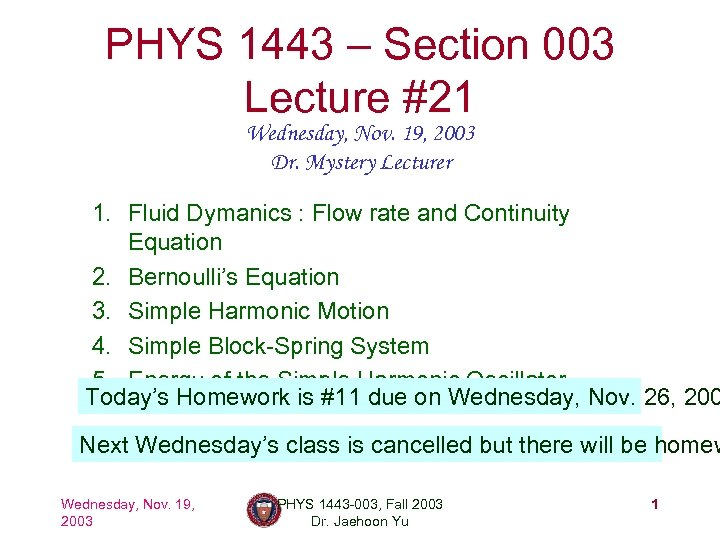PHYS 1443 – Section 003 Lecture #21 Wednesday, Nov. 19, 2003 Dr. Mystery Lecturer 1. Fluid Dymanics : Flow rate and Continuity Equation 2. Bernoulli’s Equation 3. Simple Harmonic Motion 4. Simple Block-Spring System 5. Energy of the Simple Harmonic Oscillator Today’s Homework is #11 due on Wednesday, Nov. 26, 200 Next Wednesday’s class is cancelled but there will be homew Wednesday, Nov. 19, 2003 PHYS 1443 -003, Fall 2003 Dr. Jaehoon Yu 1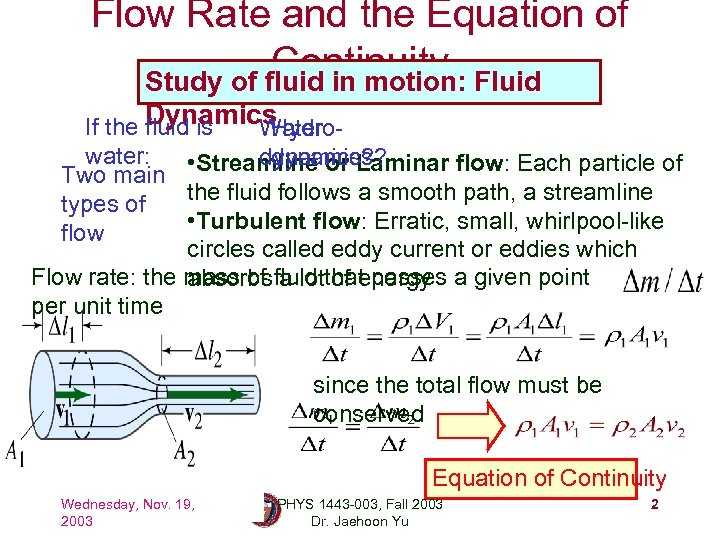Flow Rate and the Equation of Continuity Fluid Study of fluid in motion: Dynamics If the fluid is Water Hydrowater: dynamics? ? dynamics • Streamline or Laminar flow: Each particle of Two main the fluid follows a smooth path, a streamline types of • Turbulent flow: Erratic, small, whirlpool-like flow circles called eddy current or eddies which Flow rate: the mass of fluid that passes a given point absorbs a lot of energy per unit time since the total flow must be conserved Equation of Continuity Wednesday, Nov. 19, 2003 PHYS 1443 -003, Fall 2003 Dr. Jaehoon Yu 2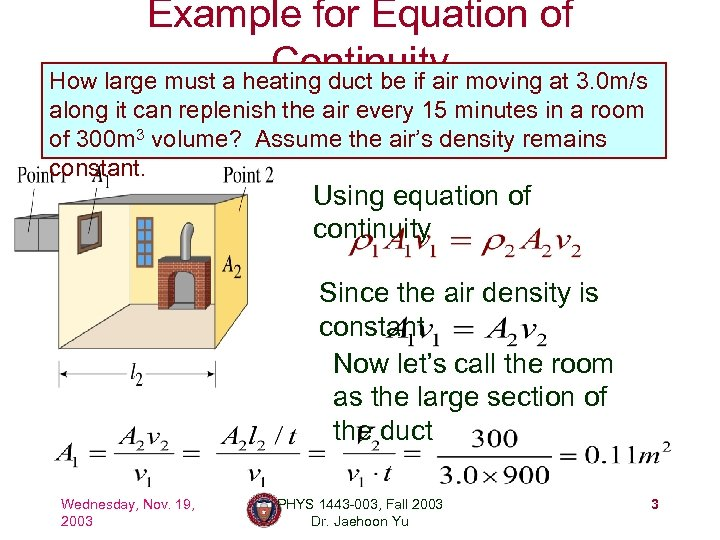Example for Equation of Continuity moving at 3. 0 m/s How large must a heating duct be if air along it can replenish the air every 15 minutes in a room of 300 m 3 volume? Assume the air’s density remains constant. Using equation of continuity Since the air density is constant Now let’s call the room as the large section of the duct Wednesday, Nov. 19, 2003 PHYS 1443 -003, Fall 2003 Dr. Jaehoon Yu 3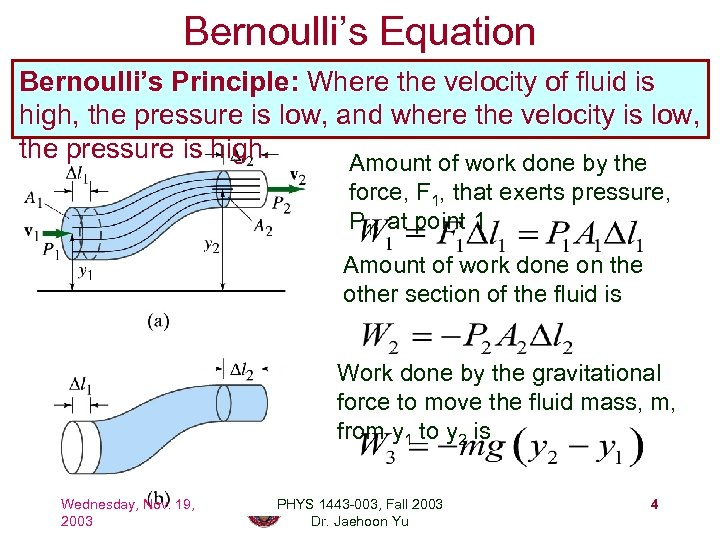Bernoulli’s Equation Bernoulli’s Principle: Where the velocity of fluid is high, the pressure is low, and where the velocity is low, the pressure is high. Amount of work done by the force, F 1, that exerts pressure, P 1, at point 1 Amount of work done on the other section of the fluid is Work done by the gravitational force to move the fluid mass, m, from y 1 to y 2 is Wednesday, Nov. 19, 2003 PHYS 1443 -003, Fall 2003 Dr. Jaehoon Yu 4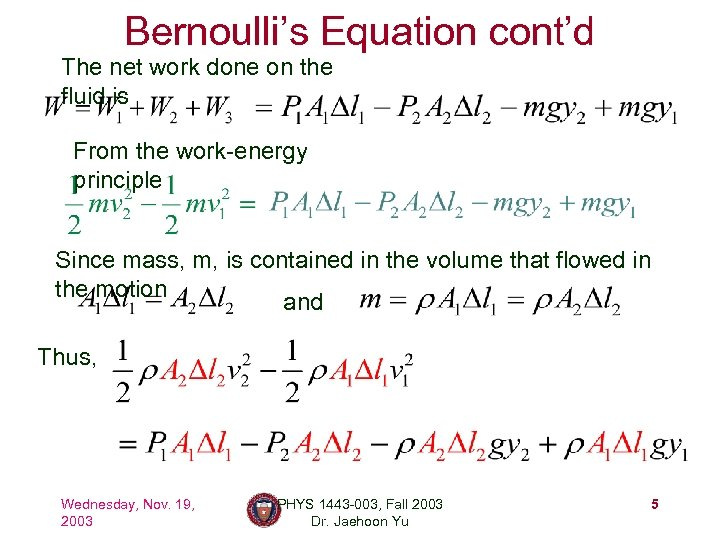Bernoulli’s Equation cont’d The net work done on the fluid is From the work-energy principle Since mass, m, is contained in the volume that flowed in the motion and Thus, Wednesday, Nov. 19, 2003 PHYS 1443 -003, Fall 2003 Dr. Jaehoon Yu 5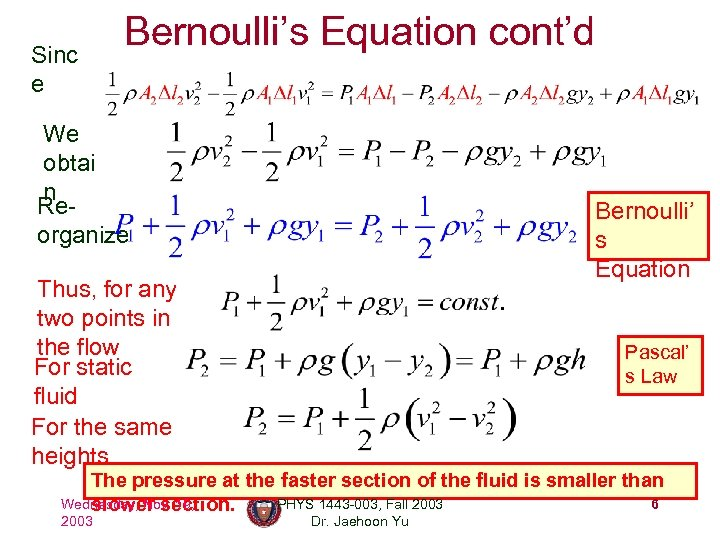Sinc e Bernoulli’s Equation cont’d We obtai n Reorganize Bernoulli’ s Equation Thus, for any two points in the flow For static fluid For the same heights Pascal’ s Law The pressure at the faster section of the fluid is smaller than Wednesday, Nov. 19, PHYS 1443 -003, Fall 2003 6 slower section. 2003 Dr. Jaehoon Yu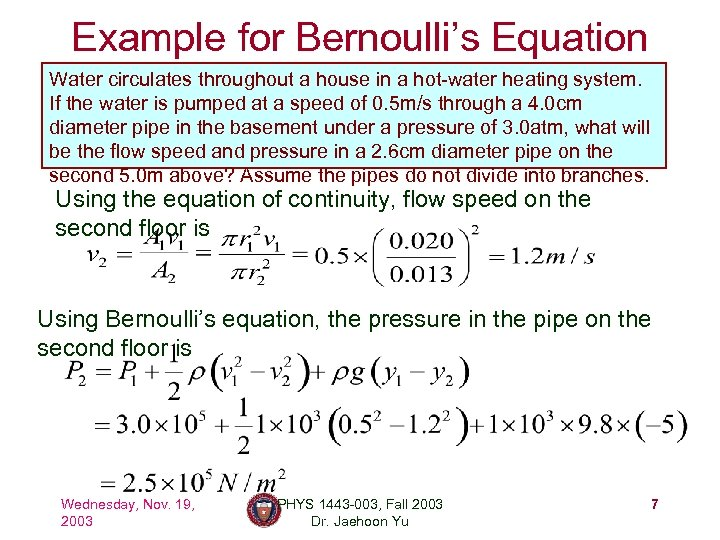Example for Bernoulli’s Equation Water circulates throughout a house in a hot-water heating system. If the water is pumped at a speed of 0. 5 m/s through a 4. 0 cm diameter pipe in the basement under a pressure of 3. 0 atm, what will be the flow speed and pressure in a 2. 6 cm diameter pipe on the second 5. 0 m above? Assume the pipes do not divide into branches. Using the equation of continuity, flow speed on the second floor is Using Bernoulli’s equation, the pressure in the pipe on the second floor is Wednesday, Nov. 19, 2003 PHYS 1443 -003, Fall 2003 Dr. Jaehoon Yu 7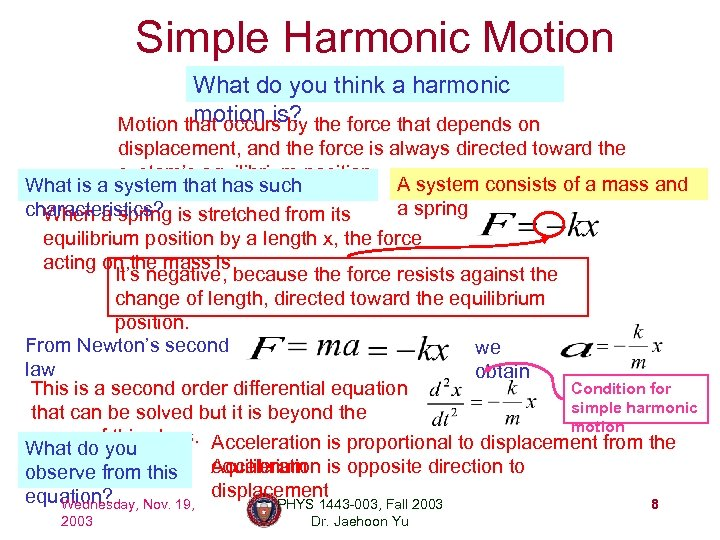Simple Harmonic Motion What do you think a harmonic motion is? Motion that occurs by the force that depends on displacement, and the force is always directed toward the system’s equilibrium position. A system consists of a mass and What is a system that has such a spring characteristics? is stretched from its When a spring equilibrium position by a length x, the force acting on the mass is It’s negative, because the force resists against the change of length, directed toward the equilibrium position. From Newton’s second we law obtain Condition for This is a second order differential equation simple harmonic that can be solved but it is beyond the motion scope of this class. Acceleration is proportional to displacement from the What do you Acceleration equilibrium is opposite direction to observe from this equation? Nov. 19, displacement Wednesday, PHYS 1443 -003, Fall 2003 8 2003 Dr. Jaehoon Yu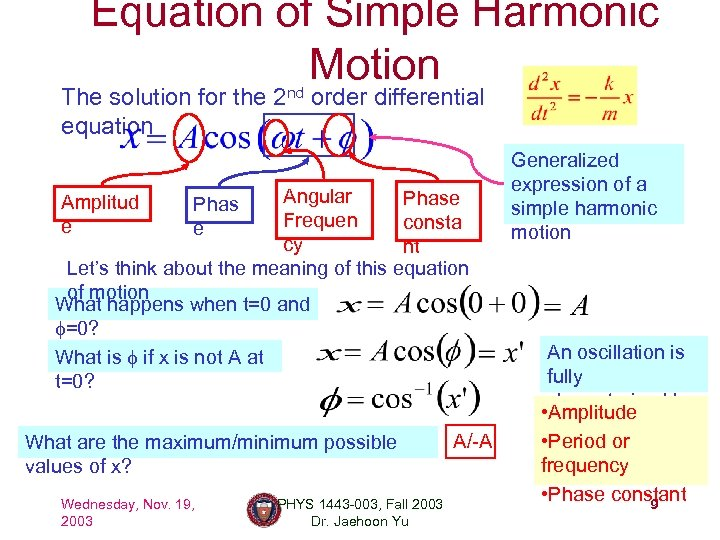Equation of Simple Harmonic Motion The solution for the 2 nd order differential equation Angular Phase Frequen consta cy nt Let’s think about the meaning of this equation of motion What happens when t=0 and f=0? What is f if x is not A at t=0? Amplitud e Phas e What are the maximum/minimum possible values of x? Wednesday, Nov. 19, 2003 PHYS 1443 -003, Fall 2003 Dr. Jaehoon Yu A/-A Generalized expression of a simple harmonic motion An oscillation is fully characterized by • Amplitude its: • Period or frequency • Phase constant 9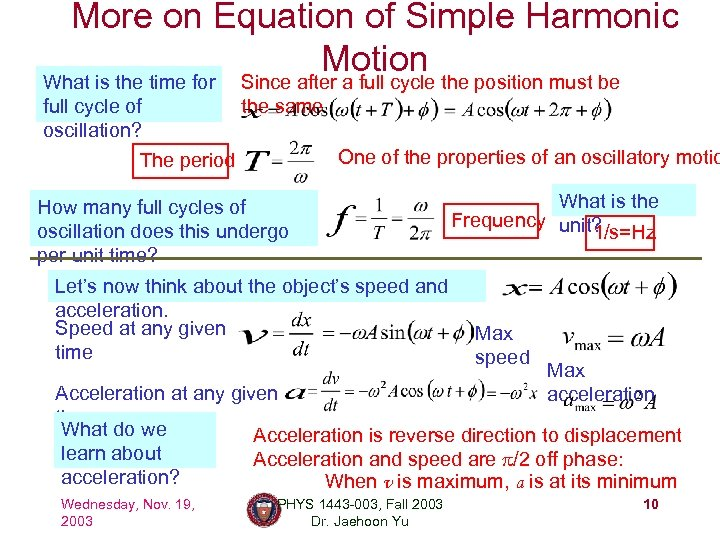More on Equation of Simple Harmonic Motion What is the time for full cycle of oscillation? Since after a full cycle the position must be the same One of the properties of an oscillatory motio The period How many full cycles of oscillation does this undergo per unit time? Let’s now think about the object’s speed and acceleration. Speed at any given time What is the Frequency unit? 1/s=Hz Max speed Max acceleration Acceleration at any given time What do we Acceleration is reverse direction to displacement learn about Acceleration and speed are p/2 off phase: acceleration? When v is maximum, a is at its minimum Wednesday, Nov. 19, 2003 PHYS 1443 -003, Fall 2003 Dr. Jaehoon Yu 10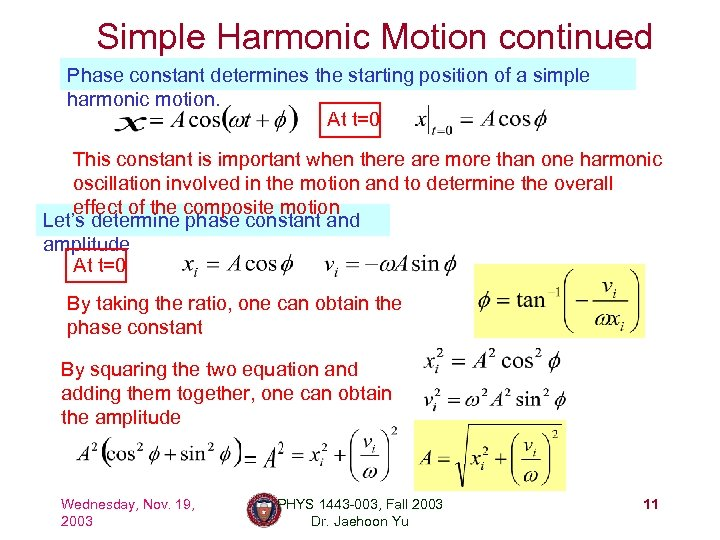Simple Harmonic Motion continued Phase constant determines the starting position of a simple harmonic motion. At t=0 This constant is important when there are more than one harmonic oscillation involved in the motion and to determine the overall effect of the composite motion Let’s determine phase constant and amplitude At t=0 By taking the ratio, one can obtain the phase constant By squaring the two equation and adding them together, one can obtain the amplitude Wednesday, Nov. 19, 2003 PHYS 1443 -003, Fall 2003 Dr. Jaehoon Yu 11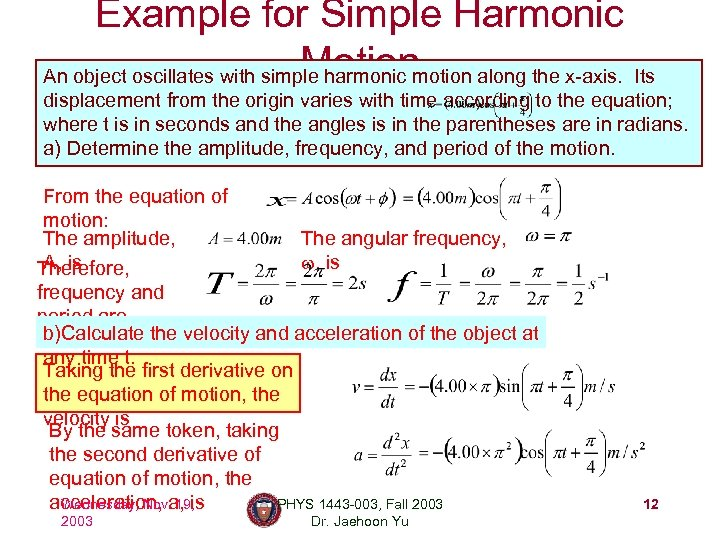Example for Simple Harmonic Motion An object oscillates with simple harmonic motion along the x-axis. Its displacement from the origin varies with time according to the equation; where t is in seconds and the angles is in the parentheses are in radians. a) Determine the amplitude, frequency, and period of the motion. From the equation of motion: The amplitude, The angular frequency, A, is w, is Therefore, frequency and period are b)Calculate the velocity and acceleration of the object at any time t. Taking the first derivative on the equation of motion, the velocity is By the same token, taking the second derivative of equation of motion, the acceleration, a, is Wednesday, Nov. 19, PHYS 1443 -003, Fall 2003 Dr. Jaehoon Yu 12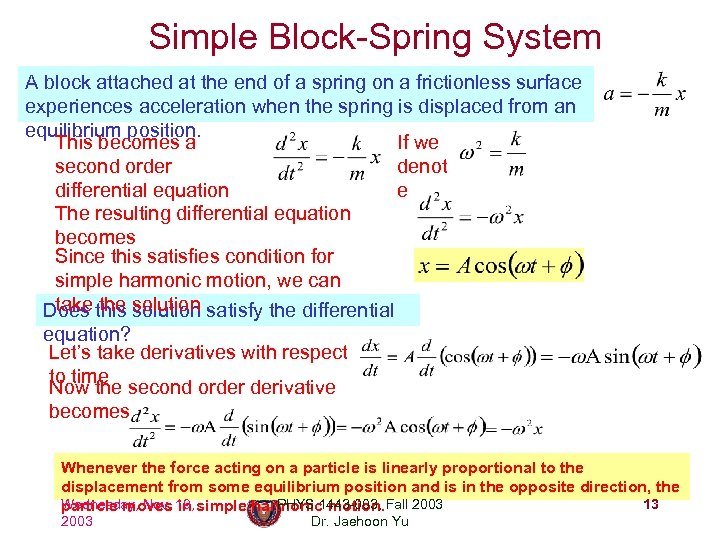Simple Block-Spring System A block attached at the end of a spring on a frictionless surface experiences acceleration when the spring is displaced from an equilibrium position. This becomes a If we second order denot differential equation e The resulting differential equation becomes Since this satisfies condition for simple harmonic motion, we can take the solution Does this solution satisfy the differential equation? Let’s take derivatives with respect to time Now the second order derivative becomes Whenever the force acting on a particle is linearly proportional to the displacement from some equilibrium position and is in the opposite direction, the Wednesday, Nov. 19, simple harmonic motion. Fall 2003 PHYS 1443 -003, 13 particle moves in 2003 Dr. Jaehoon Yu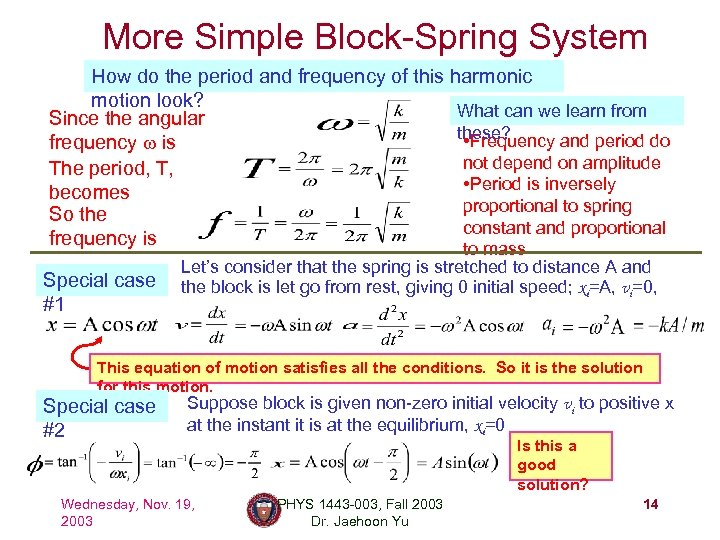More Simple Block-Spring System How do the period and frequency of this harmonic motion look? What can we learn from Since the angular these? • Frequency and period do frequency w is not depend on amplitude The period, T, • Period is inversely becomes proportional to spring So the constant and proportional frequency is Special case #1 to mass Let’s consider that the spring is stretched to distance A and the block is let go from rest, giving 0 initial speed; xi=A, vi=0, This equation of motion satisfies all the conditions. So it is the solution for this motion. Special case #2 Suppose block is given non-zero initial velocity vi to positive x at the instant it is at the equilibrium, xi=0 Wednesday, Nov. 19, 2003 Is this a good solution? PHYS 1443 -003, Fall 2003 Dr. Jaehoon Yu 14